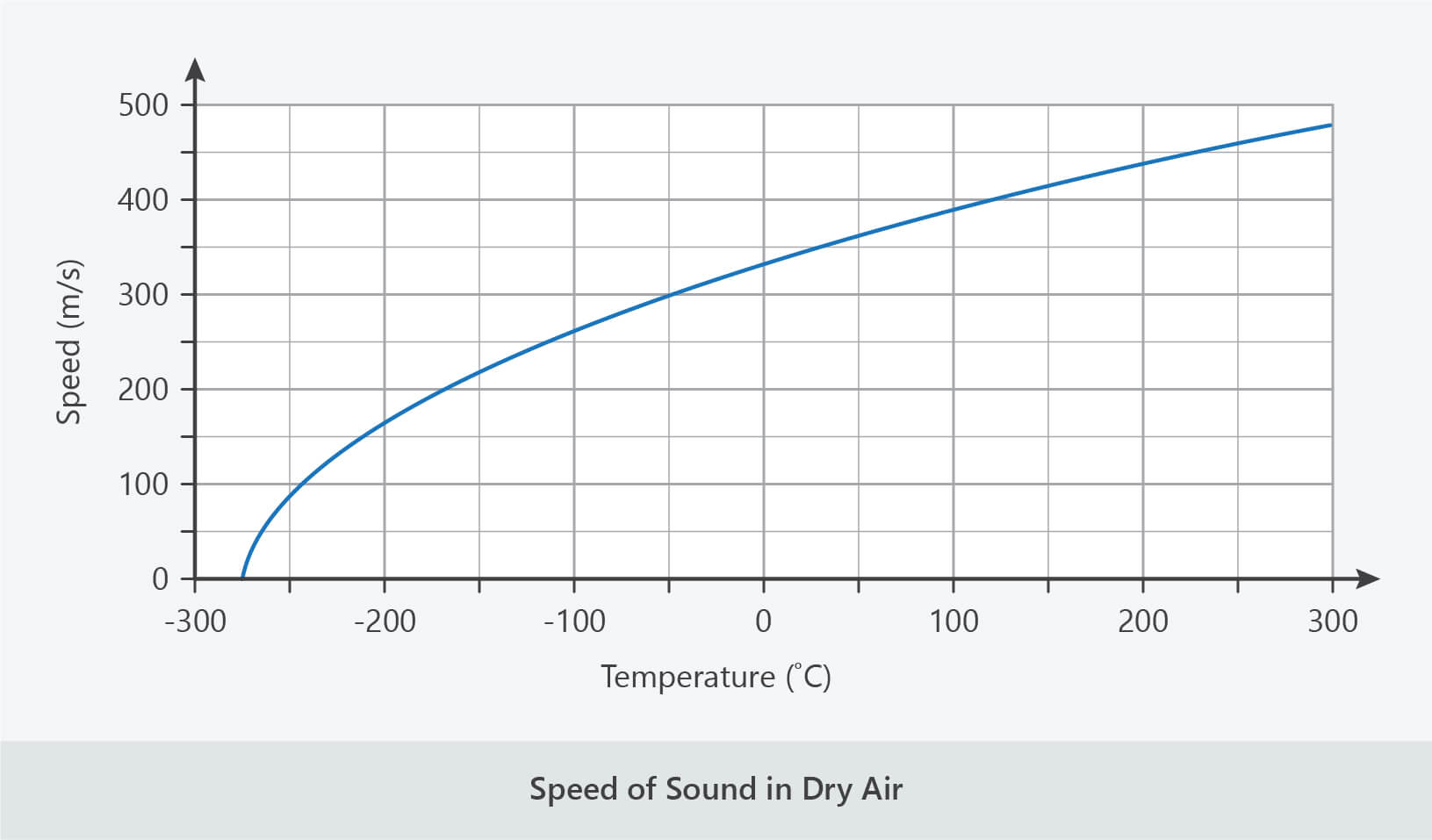BUY THE YEAR 9 PHYSICS WORKBOOK

## Speed of Sound

• The velocity (speed) of a wave is also mathematically related to distance and time, as shown in the following formulas.
• In terms of speed:• In terms of distance:• In terms of time:• We saw in the lesson ‘Waves’ that the speed of a wave depends on the medium through which it travels.
• We saw that mechanical waves, such as sound waves, travel fastest in solids and slowest in gases.
• This is because the more closely packed particles in a substance are, the more efficiently they can transfer the wave.
• Examples
• The speed of sound in air is approximately 340 m/s.
• The speed of sound in water is approximately 1500 m/s.
• The speed of sound in concrete is approximately 3000 m/s.
• The speed of sound is also affected by temperature. Particles are moving faster in hotter objects than in cooler objects, therefore sound waves can travel more efficiently through them.
• Examples
• The speed of sound in air at 0 °C is approximately 331 m/s.
• The speed of sound in air at 25 °C is approximately 346 m/s.
• The speed of sound in air at 45 °C is approximately 358 m/s.
• The speed of sound in air is also affected by the composition and density of the air, including the amount of water vapour.
• For example, sound travels slightly faster in humid air than in dry air.The speed of sound varies with temperature.